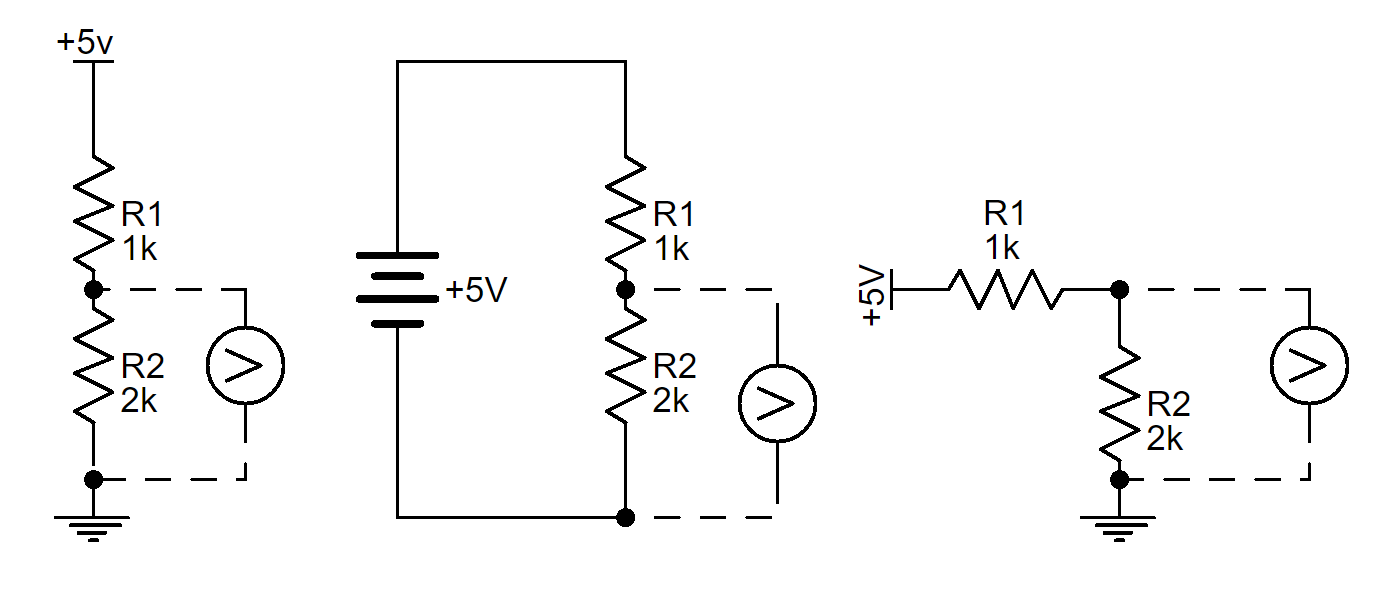# Learning Electronics - Voltage dividers

In this post I continue my reading and experimentation into electronics fundamentals and attempt to get my head around voltage dividers (also known as ‘potential dividers’) using resistors. While this post is mainly a set of notes for my own reference while learning electronics, I hope this might be of use to others. I should point out that as I am a learner, some details may be incorrect, for which I can only apologise.

Voltage dividers or ‘potential dividers’ are useful for various applications, particularly logic-level shifting and reference voltages for sensors and ICs, a voltage divider can be used along with an op-amp to create a light or darkness detector, for example.

It should be noted that voltage dividers are not suitable for providing a regulated voltage supply to power circuits, ie: powering an Arduino-type project from a 12v supply. If you do need a reliable regulated voltage, I’d recommend using a monolithic linear voltage regulator such as the LM317 (variable output) or 5v L7805 which can be bought very cheaply from eBay or from your favourite parts supplier.

In the image below, three possible schematic layouts for a voltage divider circuit are shown with the two resistors, R1 and R2 labelled. I would often see the third image, a resistor connected straight to ground and wondering what it did. While the three examples look quite different, they each do exactly the same thing:Also in the above image, I included a voltmeter represented by the circled ‘V’ with the dashed lines representing the probe leads. In this case, measuring the divided voltage with the red probe at the junction of R1 and R2, while the black probe would be connected to ground.

### Potentiometers are potential dividers!

It’s worth noting that potentiometers (variable resistors) work on the same principle as a 2-resistor divider, if you imagine the wiper (the middle pin) as the measuring mid-point separating the two ‘resistors’ in series.

### The equation

I love learning electronics, but find some of the theoretical, math-heavy stuff extremely off-putting, even moreso with the use of those Greek symbols! Luckily, the equation for calculating the output of the voltage divider is very simple and memorable:So, let’s say I have a 5v supply voltage and have a 1kΩ and 2kΩ resistor, the equation for the divided voltage will be `5 x (2000 / (1000 + 2000))`, which equals 3.33v.

### Three simple rules

In addition to remembering the equation above, these three rules may help deciding on which combination of resistors to use:

• If the values of R1 and R2 are equal, V(out) will be half the supply voltage
• If R2 is a much higher value than R1, then V(out) will be closer to the supply voltage
• If R1 is a much higher value than R2, then V(out) will be tiny compared to the supply voltage

### Putting the theory to the test

Knowing how a voltage divider works and how to calculate the output voltage, I then decided to run several tests with differing combinations of resistors and using my multimeter to measure the divided voltage. I did a rough caluclation on paper before measuring. By the way, for non-standard values I used two resistors in series (2x2k for 4k, for example).

V(in)R1 Value (Ω)R2 Value (Ω)V(out)
5V1k1k2.56V
5V1k10k4.6V
5V10k1k454mV
5V1k2k3.3V
5V2k4k3.3V
5V2k1k1.67V

Notice that 1k/2k and 2k/4k result in the same voltage out! All that is required is that the two resistors are in the correct proportion.

As you can see, it is now very easy to get a 3.3v signal from a typical 5v supply just by using two resistors. I wonder if substituting one of the resistors for a potentiometer could result in specific variable voltage ranges, I’m not sure what application that could have, but it would be interesting to experiment with all the same.